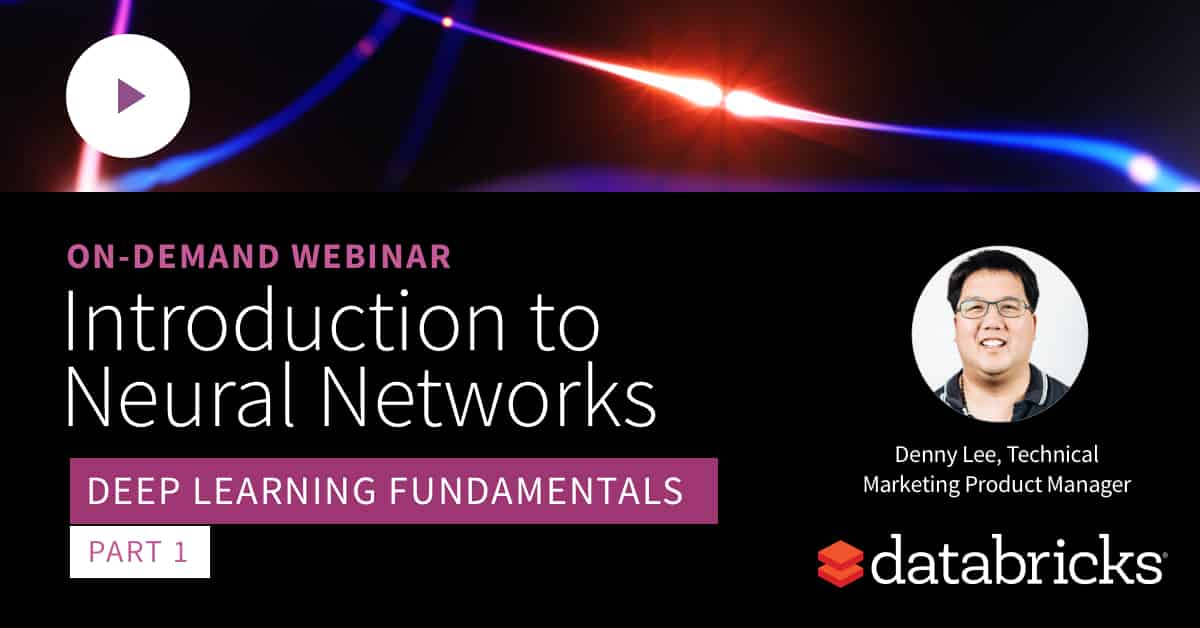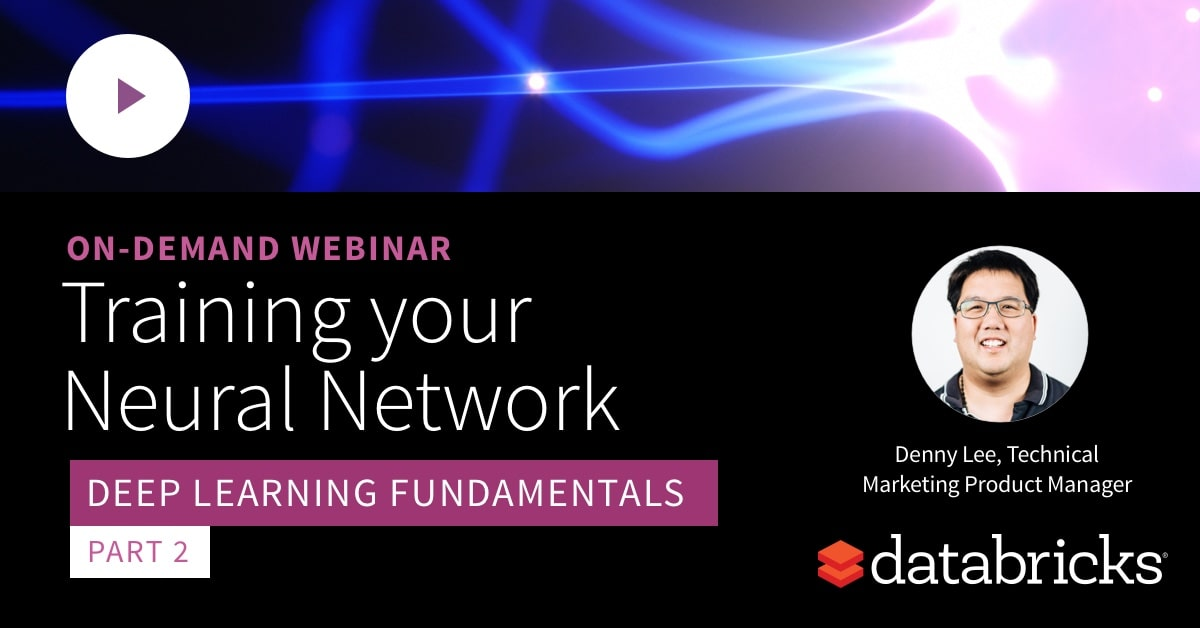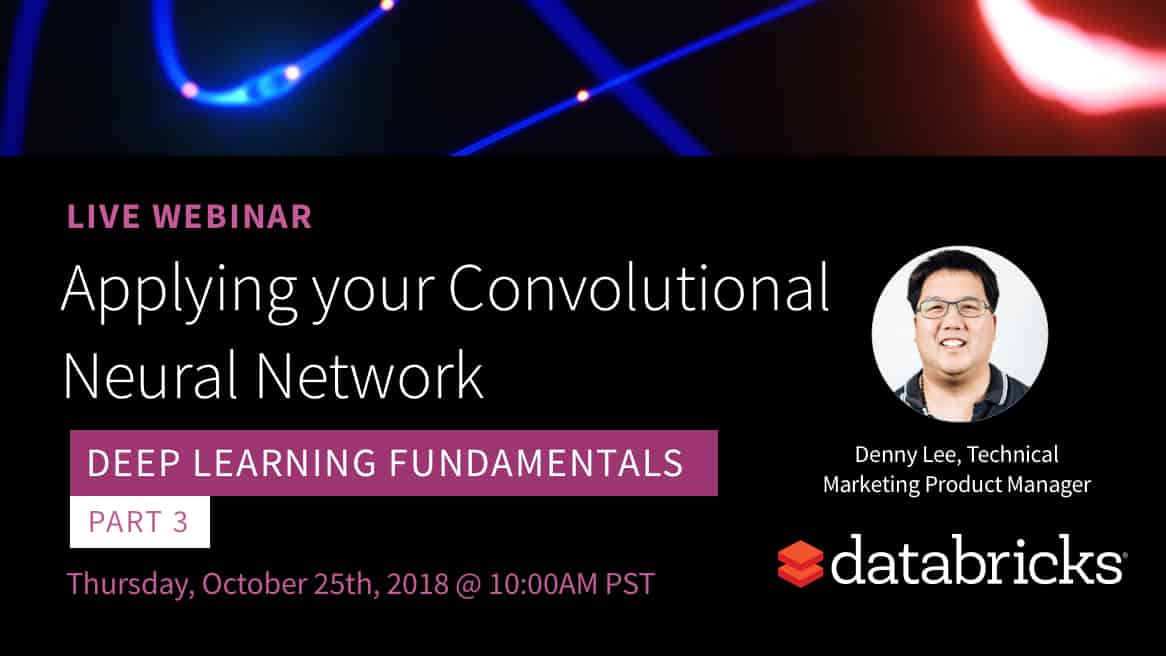Deep Learning - Databricks

Deep Learning Fundamental Series

This webinar series covers deep learning fundamentals with a focus on Keras and TensorFlow.

Deep Learning has shown tremendous success, but what makes it so special? What are neural networks, and how do they work? What are the differences between popular Deep Learning frameworks like Keras or TensorFlow, and where should you start?Part 1 – Introducing Neural Networks

Understand the relationship between neural networks and simpler ML models, and what gives neural networks their expressive power.Part 2 – Training your Neural Network

Discuss how to effectively train your neural network by reviewing the principles behind activation and loss functions, batch sizes, data normalization, and validation datasets.Part 3 – Applying your Convolutional Neural Network

Learn how convolutions works, discuss some of the ImageNet architectures and see how to apply a convolutional neural network using the ImageNet scenario.